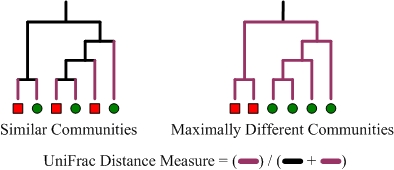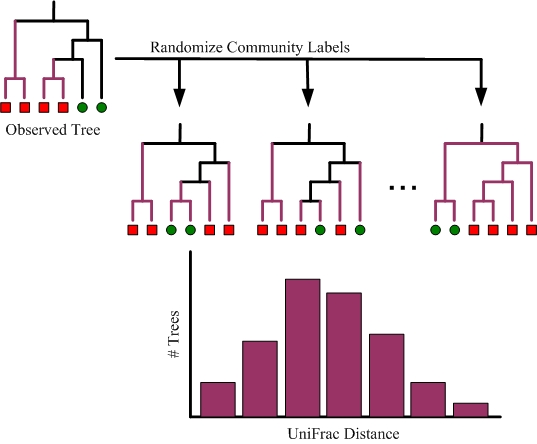# Unweighted UniFrac algorithm

The unweighted UniFrac algorithm is an attempt at creating a phylogenetic extension of the Jaccard index, which defines the distance between a pair of communities as the proportion of evolution that is unique to one community or the other Lozupone and Knight (2005). It attempts to capture the notion that processes such as adaptation and genetic drift will cause the amount of evolution unique to a community to increase as pairs of communities become increasingly genetically isolated due to diverging habitats, function, or spatial proximity. The measure explicitly considers a phylogenetic tree in order to calculate the fraction of branch length that leads to descendants from a single community.

In the examples below, there are two microbial communities identified by red squares and green circles. The branches colored purple are those that lead to descendants from a single community. UniFrac measures the amount of evolutionary divergence between these two communities by dividing the length of the purple branches by the total branch length of the tree. The example on the left shows two communities which have little evolutionary divergence and therefore a relatively low UniFrac distance. In contrast, the example on the right shows two communities that are maximally different as their lowest common ancestor is the root of the tree.Formally, the measure is calculated using the following formula, where $$N$$ is the number of nodes in the tree, $$l_i$$ is the branch length between node $$i$$ and its parent, and $$A_i$$ and $$B_i$$ are indicators equal to 0 or 1 as descendants of node $$i$$ are absent or present in communities A and B respectively.

$u = \frac{\sum_{i=1}^{N} l_i | A_i - B_i |}{\sum_{i=1}^{N} l_i \max(A_i, B_i)}$

UniFrac can be applied to multiple communities by first constructing a single tree consisting of all sequences. The distance between any pair of communities is calculated by pruning the tree so it contains only sequences from these communities. (This is implicit in the formula given above because terms for nodes not appearing in either of a pair of communities are zero and do not affect the result.)

A random (Monte Carlo) permutation test can be performed to test whether or not the distance between two communities is greater than expected by chance alone. As illustrated below, this is done by permutating the assignment of community labels to sequences while keeping the tree constant. The reported p-value is the proportion of random permutations that result in a UniFrac distance greater or equal to the observed data.